Home Articles Modelling Dynamic Weight of a Route for 3D Navigation

# Modelling Dynamic Weight of a Route for 3D Navigation

Ivin Amri Musliman 1 , Alias Abdul Rahman 1 and Volker Coors 2

1 Dept. of Geoinformatics, Faculty of Geoinformation Science & Engineering, Universiti Teknologi Malaysia
81310 Skudai, Johor, Malaysia.
[email protected]
[email protected]

2 Faculty of Geomatics, Computer Science and Mathematics, Hochschule für Technik
Schellingstr.24, 70174 Stuttgart, Germany
[email protected]

Abstract
Currently, most of shortest path algorithm used in GIS application is often not sufficient for efficient management in time-critical applications such as emergency response applications. It doesn’t take into account dynamic emergency information changes at node/vertex level especially when applying in emergency situations such as large fires (in cities or even in buildings), flooding, chemical releases, terrorist attacks, road accidents, etc. In this paper, an approach for finding shortest path or route in a dynamic situation for indoors and outdoors using Single Sink Shortest Path (SSSP) routing algorithm is proposed. This paper discusses the construction of 3D dynamic networks, as well as the corresponding algorithm that can be used for 3D navigation in a 3D GIS environment. The aspect of this research that distinguishes it from other work on the dynamic shortest path problem is its ability to handle “multiple heterogeneous modifications”: between updates, the input graph is allowed to be restructured by an arbitrary mixture of edge insertions, edge deletions, and edge-length changes.

The paper is organized in three general parts. The first part discusses the 3D navigation model, dynamic weight and its functional requirements. The second part presents the 3D dynamic network model and elaborates on the possible solution using SSSP routing algorithm and its implementation. Final discussion on recommendations for future research concludes the paper.

1.0 Introduction
Way finding or routing has been always used by common people in navigating from one place (of origin) to another (destination). And most of them are in two dimensional routing or network. In orthogonal concept, two-dimensional (2D) or three-dimensional (3D) routing uses planar or non-planar graph and spatial extend, where the third dimension is used to calculate the weights for the edges in the graph. Usually routing is done in a planar graph embedded in 2D space. Sometimes it is extended to non-planar graphs to model bridges, etc. but still embedded in 2D space. For example, if the network model type is planar (directed) graph, where the node as 0D coordinate (x, y) in 2D space, therefore objects such as bridges, flyover, etc. cannot be modeled. While for non-planar (directed) graph, where node as 0D coordinate (x, y) in 2D space, objects such as bridges, flyover, etc. can be modeled but street length cannot be derived from the model (directly). And as for 3D space (which is the target of this research), objects such as bridges, flyover, etc. can be modeled in a non-planar (directed) graph, with node as 0D coordinate (x, y, z). Street length can only be derived from the model, if arcs are close to original street geometry, which is not necessary for routing. For routing, a node is necessary at each junction etc… A slope road with no junction still can be modeled with two nodes and one arc for routing purposes even if it is long and has a lot of serpentines as usual in the mountains but this is the geometry part of the network.

Routing in 3D is almost impossible at the beginning, but with the emergence of new models, concepts and improved algorithms for way finding and navigation together with the development of communication and positioning technologies, it has made researchers expand the focus this research area. Modeling a route or network with dynamic behavior requires new data structures to represent the complexities of the networks and by applying different type of network algorithms (e.g. shortest path algorithm) based on real time input behaviors, navigating in such network is possible and enhances its potential for 3D navigation in 3DGIS environment.

Currently, research efforts have been initiated by researchers to introduce concepts for routing in 3D-GIS (Ivin et. al. 2006, Zhu et. al. 2006) and even for evacuation strategies (Shi and Zlatanova 2005) with several types of developments and approaches. Most of the discussed researches were based on transportation data model (Zhu et. al. 2006, Liu et. al. 2005, Fischer 2004) and others are focusing on disaster and emergency management (Shi and Zlatanova 2005, Zlatanova et. al. 2005, Zlatanova and Holweg 2004). What is missing is the mapping of the real world or a 3D model to the weights or costs of an edge in a network graph.

Before continuing, let us describe some notations and define the basic shortest path problem. A network is a graph, G = (V, E) consisting of set of nodes (vertex) V, with the collection of network nodes g =V|; g =V1, V2, V3,…Vnand a spanning set of directed edges E with h =E|; h =(V1, V2), (V2, V3), …, (Vn, Vn)|. Each edge is represented as a pair of nodes, from node i to node j, denoted as (i, j). Each edge (i, j) has a weight, associated with a numerical value, Wij, which represents the distance or cost of the edge (i.e. Wij : 5). In this paper, we assume that two-directional travel between a pair of nodes i and j is represented by two different directed edges (i, j) and (j, i). Given a network G = (V, E) with known edge weight (distance) Wij for each edge (i, j) subset to E, the shortest path problem is to find the shortest distance path from a source node s to a specific node in the node set V. This is a simple way of finding shortest path in a network, with a static node(s). There are some cases where behavior (i.e. location, weight & related surrounding attributes) of one or more nodes are dynamically change due to unforeseen events in the future, either along or surround the route to the destination.

In this paper, the study focuses on model construction of dynamic weight of a network for 3D navigation that can be used in any navigation situation, indoors and outdoors or both. In a dynamic navigation situation, the main question one would ask is what will happened to the weight of an edge; Wij, of the non-planar graph over dynamic changes e.g. time, current situation, etc. on the route itself?. Which model is suitable for each of the dynamic changes for the route?. For example, in the case of fire in a certain floor of a building, unknown burned ceiling suddenly collapses and blocks existing escape path, so a new escape path will be selected real time due to dynamic changes on the route. This also applies to a case where an emergency vehicle routing is re-routed real time in a city due to congested network traffic flow occurred from cars accident or oil spills in peak hour and etc.. This paper is divided into 5 sections. Following this introduction, Section 2 reviews the 3D navigation model, dynamic weight and its functional requirements; Section 3 introduces the 3D dynamic network model; Section 4 presents the possible solution for routing algorithm and its implementation; and finally the conclusion and recommendations for future research are given in Section 5.

To improve current data models and construct new data models/standards, studying the concept and logic model is the base. GDF, which became an ISO standard in early 2004, is more a common concept and logic model of road networks and road related information for navigation service. And its physical model can act as exchangeable format for navigation data. However, SDAL are more physical data models. The concept and logic data model study based on GDF is meaningful.

Graph data structure allows an edge to connect to other edges only at its end points. Fohl et. al. (1996) suggested a way to adapt the lane-based network to work with the existing routing algorithms by representing a lane segment with a series of small edges so that lane changing can be made to adjacent lanes at any vertex along the series.

2.1 Concept of Dynamic Weight
In dynamic graph with time dependent G{V(t), E(t)} a node/vertex may be removed or added, depends on the dynamic changes that occurred real-time on the graph especially in buildings. Weight of the edges is also changes over time. For example, in an emergency situation, e.g. fire in a specific floor in a building, elevators will be assigned as a dynamic node/vertex (see Fig. 1). In case of a fire incident, it is advised to use the stairs instead of elevators. Considering the complexity of modern buildings and the great numbers of people that can be inside the buildings, it is rather difficult to organize a quick evacuation.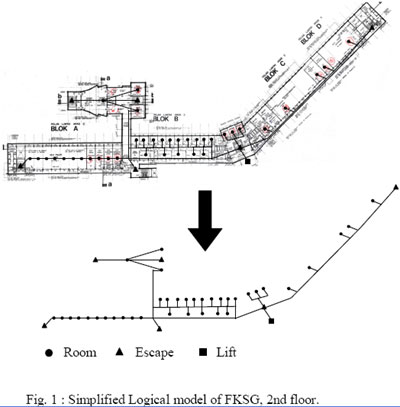Room Escape Lift
Vehicle routing problem. In this case, destination node is fixed, whereas the start node would be dynamically located anywhere on the map. For example, call for a taxi; a person calls a taxi company and gives his/her location to the operator. Then the operator will searched and dispatched the nearest taxi to the given location and informed the caller the estimated time of arrival of the taxi. With a dynamic factor such as road traffic taking into account, the assigned taxi might not be able to arrive at the specific location in time. Other available taxi (second nearest) will then be notified, and the process goes on until a taxi reached the caller. Algorithm that will be used for this type of problem will have one-to-many type Therefore this type of case is much easier than the following problem.

Rescue operation planning. Would the model be able to assign each rescuer to survivor(s) in a rescue operation and evacuation process with known or unknown number of survivor in case of a fire situation? The model of a building can either be an abstraction of a building is represented with polygons in 3D space (Zlatanova et. al 2004) which is likely geometry or topology model, or a logical model, which represents the connections between the rooms. The rooms and important crossings are represented with nodes; the paths are represented as links between nodes

The requirements of real-time, more accuracy and individuation for dynamic navigation can be met in the situation. There are at least two elements to realize dynamic navigation in vehicle navigation. Firstly, is the data that reflects the real-time traffic information. Secondly, the effectiveness and highly precise algorithms of short time traffic prediction. Dynamic navigation for vehicle navigation relies on the real-time traffic data (Liu et. al. 2005). Zhu et al. (2006) have addressed a model for emergency routing for escape plan. In this model, it emphasis on multi-dimensional and dynamic routing algorithm for vehicle emergency. The algorithm is based on the functional requirement analysis of 3D vehicle emergency routing. Detailed discussion about the efficiency analysis of shortest path algorithms can be found in Zhan and Noon’s (1998) research.

Navigating indoors and outdoors virtually need to have ‘seamless’ continuation between them. By using simulation (Jafari et. al 2003), it may improve the dynamic understanding of navigation . Some information will be temporarily unavailable in some special situation such as poor weather or technical problem, therefore the use of simulation information will provide continuous and dynamic support. Simulation of environment scenarios under different assumptions, may minimize the costs and threats with a predicted manner.

2.2 Functional Requirements
From the user’s perspective, emergency routing and navigation relate to life threatening and asset damaging events. In contrast to other traditional ‘shortest’ path applications (such as traveling salesman problems), emergency vehicle and indoor evacuation routing primarily calls for minimum travel time rather than minimum travel distance, or other considerations.

3.0 3D Dynamic Network Model
In short, the overall process of building a 3D dynamic network can be shown briefly in three steps. Firstly, from planar and non-planar networks to 3D networks, the ambiguous situation of under/overpass and network overlay in the 2D graph can be clarified. It improves the abilities of visualization and effective and comprehensive data integration. Secondly, by moving from a static network to a dynamic network, real-time information about the vehicle and other events can be directly integrated into the routing process. Lastly, after optimization, the unnecessary vertices are eliminated and the total number of vertices is greatly reduced, thus improve the ability of rapid response.

Distances can be calculated be distance between 2 node coordinates. Elevators have to be models as vertex per floor with connecting edges. Stairs have to be models as edges. It can happen that two parallel stairs connect the same nodes. This means that E is no set any more. In a set, each element is unique! But in this case two nodes are connected by two distinguishable edges E1 = (Vi,Vj) and E2 = (Vi,Vj); this is similar to a model with lanes. Parallel edges are allowed.

3.1 Anticipated Routing Algorithm Implementation
As mentioned before the routing graph might change over time due to specific events. As we will see, the two most important changes are increasing and decreasing the costs of an edge and inserting a new edge into the graph. Other events can be modelled based on these two operations. Inserting a new edge e=(v,w) to a graph can be considered as decreasing the costs c(e) from 8 to a value c. Deleting an edge e=(v,w) is similar to increase the costs of an edge to 8. Deleting a vertex v can be done by deleting all edges that are incident to v. Inserting a vertex is trivial as long as no edge is connecting it with the rest of the graph. These connecting edges will be inserted using the inserting edge algorithm. Table 1 below described when it is required to delete, insert or modifying an edge or vertex.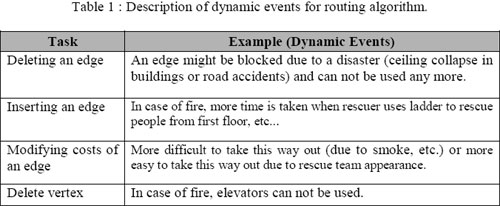Deleting an edge An edge might be blocked due to a disaster (ceiling collapse in buildings or road accidents) and can not be used any more.

Inserting an edge In case of fire, more time is taken when rescuer uses ladder to rescue people from first floor, etc… Modifying costs of an edge More difficult to take this way out (due to smoke, etc.) or more easy to take this way out due to rescue team appearance. Delete vertex In case of fire, elevators can not be used. In order to find the shortest way out of a building in case of an emergency from a given location or in traffic jams, the Single-Sink Shortest Path problem (SSSP) has to be solved for the dynamic graph G. Under the assumption that every edge e=(v,w) in the graph has positive costs c(e)>0, the problem can be solved by Dijkstra algorithm. If the structure of the graph changes due to some event, the whole algorithm has to be run again even if the changes do not have any effect on the result. The Dijkstra algorithm can be considered as batch processing on a given input graph G and sink Vertex s. If the data input is changed, the algorithm as to be run again. In this chapter we propose an incremental approach to deal with the changes of the graph structure. Changes in the graph structure are usually local changes. Once the SSSP problem is solved for the given input (G, v), only a small part of the solution has be recalculated due to the event. This incremental approach is usually much more efficient. For a detailed analysis of complexity of this kind of incremental algorithms can be found in Ramalingam and Reps’s (1996) research.

3.2 Incremental SSSP Algorithm
The input of the SSSP problem is a graph G (V, E), a cost function c: E à R+ and a sink vertex v. The shortest distance dist(w) from v for every vertex w V should be computed. Dijkstra algorithm will solve the problem. However, G changes over time as discussed before. We are interest in an incremental algorithm that handles these changes without solving the SSSP problem for the whole graph again.

Under the assumption that the shortest path from v to w is unique for every vertex w V, the resulting shortest paths build a spanning tree of G. This spanning tree ST consists of edge e E that is used in at least on shortest path. Thus, e=(t,u) ST if and only if dist(u) = dist(t) + c(e). In general, a shortest path might not be unique. It might be that a vertex could be reached on two different paths with the same costs. In this case, the resulting shortest paths do not build a spanning tree any more but a directed acyclic graph (DAG).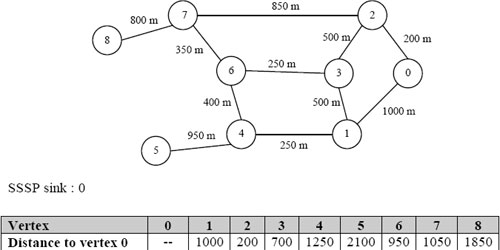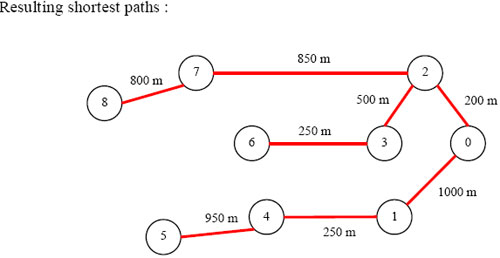3.2.1 Deleting / increasing costs of an edge
Increasing costs c(e) of an edge e=(t,u) or deleting this edge only has an effect to the solution of the SSSP problem if the edge e is part of an existing shortest path, thus e ST. In that case, all nodes that use the modified edge in their shortest path might be affected by this event. These nodes are the successors of node t in ST. Other nodes can not be affected because the costs for e are increasing. To recalculate the shortest paths form the sink to the affected vertices we simplify the graph as follows. All vertices that are not affected by the event will be treated as a single vertex. Each edge e=(x, y) of the affected vertices x to one vertex y that is not affected will get a new weight: cs’(e)= c(e) + dist(y). The SSSP problem is solved again with this simplified graph. For example; Delete Edge e=( v0, v1) Affected vertices: v1, v4, v5. Not affected vertices: v0, v2, v3, v6, v7, v8.

3.2.2 Inserting / decreasing costs of an edge
Decreasing costs c(e) of an edge e=(t,u) or inserting a new edge. A vertex w is affected by this event if the new edge e enables a shorter path from v to w with distnew(w) distold(w). The new edge e has to be part of this new shortest path. The length of the new shortest path is given by distnew (w) = dist(t) + c(e) + dist(u,w) where dist(u,w) is the length of the shortest path between u and w.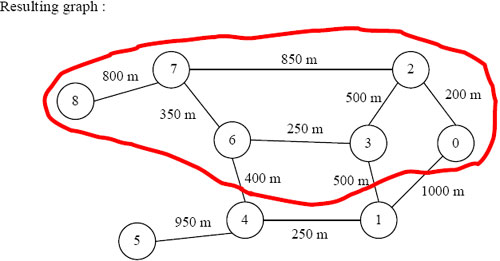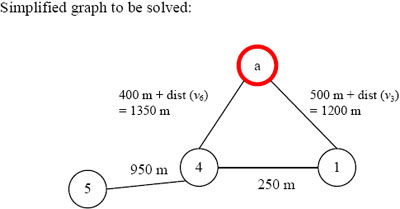The algorithm works similar to Dijkstra algorithm. In a batch implementation of Dijkstra algorithm all adjacent vertices vi of a vertex x are adjusted if dist(x) + c(x, vi) dist(vi) where dist(vi) is the shortest distance so far. In the incremental version of the algorithm if edge e=(t,u) is inserted into G or the costs of the (already existing) edge is decreased, it has to be checked if dist(t) + c(t,u) < dist(u) or dist(u) + c(t,u) < dist(t). If this is the case, the algorithm continues with the affected vertex similar to the batch implementation of Dijkstra algorithm. Otherwise, the inserted edge does not change the shortest paths. 3.3 Implementation of the Incremental SSSP
This section describes the implementation of the incremental SSSP algorithm using 2D datasets (of road network). The interface was developed using new ‘classes’ within Visual Basic 6.0 environment. Figure 2 illustrates the shortest path from A (vertex 1) to B (vertex 57) using standard Dijkstra algorithm. On the other hand, Figure 3 shows a new route derived from the implemented algorithm based on a dynamic event occurred in one of the edges along the original shortest path route from A to B. The dynamic event occurred at one edge that consists of vertex 18 as source and vertex 29 as destination. While the dotted line represents the original shortest path route.

1 ae 4 5 950 m 400 m + dist (v6) = 1350 m 250 m 500 m + dist (v3) = 1200 m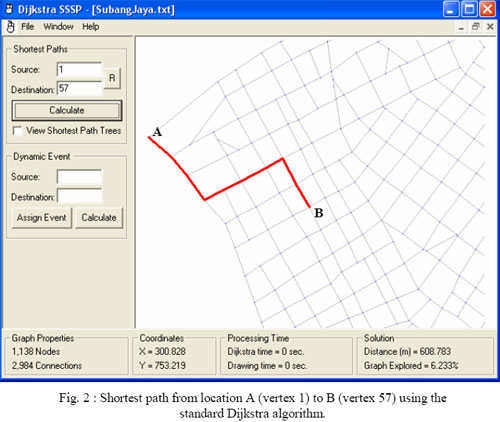4.0 Conclusion and Recommendations
This paper suggested a new concept of calculating shortest path routes that supports dynamic changes information. The initial results are shown in section 3.3. Based on the concepts given in this paper, implementation of the dynamic indoor evacuation and shortest path route calculation algorithm for vehicle will be carried out. Also, in simulating environment scenarios under different assumptions, further works need to be looked at and addressed from 2D to 3D.

References

• Dueker, K. J., & Butler, J. A. 1997. GIS-T Enterprise Data Model with Suggested Implementation Choices. [Electronic Version]
from
• Fischer, M. M. 2004. GIS and Network Analysis. [Electronic Version]
from
• Fohl, P., Curtin, K. M., Goodchild, M. F. and Church, R. L., 1996. A non-planar, lane-based navigable data model for ITS. In M.J. Kraak and M. Molenaar (eds.) Proceedings, Seventh International Symposium on Spatial Data Handling, Delft, August 12-16, pp. 7B.17-7B.29
• G. Ramalingam and T. Reps, 1996. On the Computational Complexity of Dynamic Graph Problems, Theoretical Computer Science, Vol 158 / 1 & 2. [Electronic Version] from https://citeseer.ist.psu.edu/cache/papers/cs/32474/http:zSzzSzwww.cs.wisc.eduzSzwpiszSz paperszSztcs96a.pdf/ramalingam96computational.pdf
• GDF4.0 Manual. Available at _geographic_data_files.htm or . com/writegdf/GDF4_Specs.zip
• Goodchild, M.F., 1998. Geographic information systems and disaggregate transportation modeling. Geographical Systems 5(1–2):19–44 Ivin Amri Musliman, Alias Abdul Rahman and Volker Coors, 2006. 3D Navigation for 3DGIS – Initial Requirements. Innovations in 3D Geo Information Systems, Springer: pp. 125-134
• Jafari, M., Bakhadyrov, I., & Maher, A. 2003. Technological Advances in Evacuation Planning and Emergency Management: Current State of the Art [Electronic Version] from
• Liu Yuefeng, Zheng Jianghua, Yan Lei, Xu Yiqin, 2005. Study on the real time navigation data model for dynamic navigation. IGARSS ’05 Proceedings. Geoscience and Remote Sensing Symposium, 2005. [Electronic Version] from https://ieeexplore.ieee.org/iel5/10226/32596/01525224.pdf
• NavTech. Available at https://www.navteq.com/sdalformat/ Shi Pu and Sisi Zlatanova, 2005. Evacuation Route Calculation of Inner Buildings. Geoinformation for Disaster Management, Springer: pp. 1143-1161
• Zhan, F. B., & Noon, C. E. 1998. Shortest Path Algorithms: An Evaluation using Real Road Networks. Transportation Science 32(1): 65-73
• Zhu Qing, Li Yuan and Tor Yam Khoon, 2006. 3D Dynamic Emergency Routing. GIMInternational June 2006, Volume 20, Issue 6. [Electronic Version] from . com/issues/article/id674-D_Dynamic_Emergency_Routing.html
• Zlatanova S., Holweg D., 2004. 3D Geo-information in emergency response: a framework. Proceedings of the 4th International Symposium on Mobile Mapping Technology (MMT’2004), March 29-31, Kunming, China.
• Zlatanova S., Holweg D., Coors V., 2004. Geometrical and Topological Models for Realtime GIS. Proceedings of UDMS 2004, 27-29 October, Chioggia, Italy.
• Zlatanova S., Holweg D. and Stratakis M., 2005. Framework for Multi-Risk Emergency Responce.